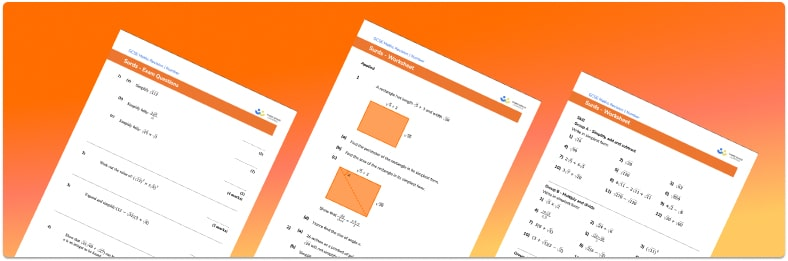# Surds Worksheet• Section 1 of the surds worksheet contains 36 skills-based surds questions, in 3 groups to support differentiation
• Section 2 contains 4 applied surds questions with a mix of worded problems and deeper problem solving questions
• Section 3 contains 7 foundation and higher level GCSE exam questions on surds
• Answers and a mark scheme for all surds questions are provided
• Questions follow variation theory with plenty of opportunities for students to work independently at their own level
• All questions created by fully qualified expert secondary maths teachers
• Suitable for GCSE maths revision for AQA, OCR and Edexcel exam boards

• This field is for validation purposes and should be left unchanged.

You can unsubscribe at any time (each email we send will contain an easy way to unsubscribe). To find out more about how we use your data, see our privacy policy.

### Surds at a glance

Surds are values written as square roots that cannot be further simplified. Surds are used to ensure very exact values are given in calculations. Surds are irrational numbers, if written as decimals, they would go on perpetually with no pattern. If written as fractions irrational numbers cannot have integer numerators or denominators.

We can multiply and divide surds. When multiplying surds, we use the rule “root a multiplied by root b = the root of a multiplied by b”. Similarly, when dividing surds we use the rule “root a divided by root b = the root of a divided by b”.

We can sometimes simplify surds by taking out factors which are square numbers. We need to keep simplifying the surd until the number under the root sign has no factors which are square numbers.

To add and subtract surds the number under the root sign must be the same. We can only add or subtract two surds with different numbers under the roots if we can simplify one or both of them so that they have the same number under the root.

Sometimes we might be asked to rationalise the denominator of a fraction. This means finding an equivalent fraction which does not have a surd in the denominator.

Looking forward, students can then progress to additional number worksheets, for example aor aFor more teaching and learning support on Number our GCSE maths lessons provide step by step support for all GCSE maths concepts.

## Do you have KS4 students who need more focused attention to succeed at GCSE?There will be students in your class who require individual attention to help them succeed in their maths GCSEs. In a class of 30, it’s not always easy to provide.

Help your students feel confident with exam-style questions and the strategies they’ll need to answer them correctly with our dedicated GCSE maths revision programme.

Lessons are selected to provide support where each student needs it most, and specially-trained GCSE maths tutors adapt the pitch and pace of each lesson. This ensures a personalised revision programme that raises grades and boosts confidence.

Find out more